# Q3: Determine the vertical and horizontal displacement of joint A for the truss shown in Fig....

Q3: Determine the vertical and horizontal displacement of joint A for the truss shown in Fig. (3), each bar is made of steel and has the cross-sectional area of 400mm?. Take E = 200 GPa. Use the method of virtual work.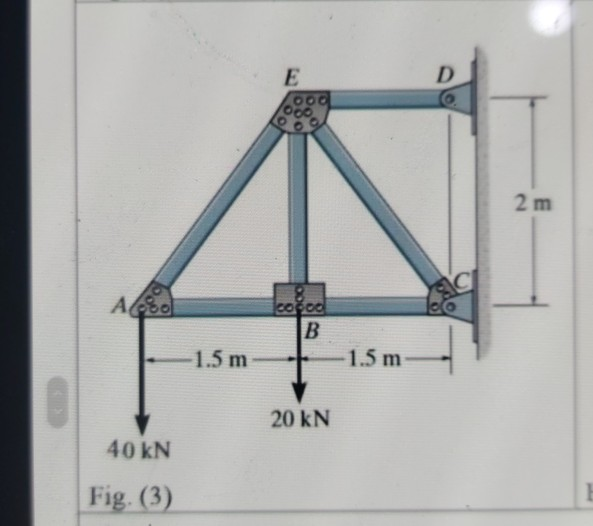E D 00. 2 m СІ Ao cocoa B 1.5 m 1.5 m 20 KN 40 kN Fig. (3)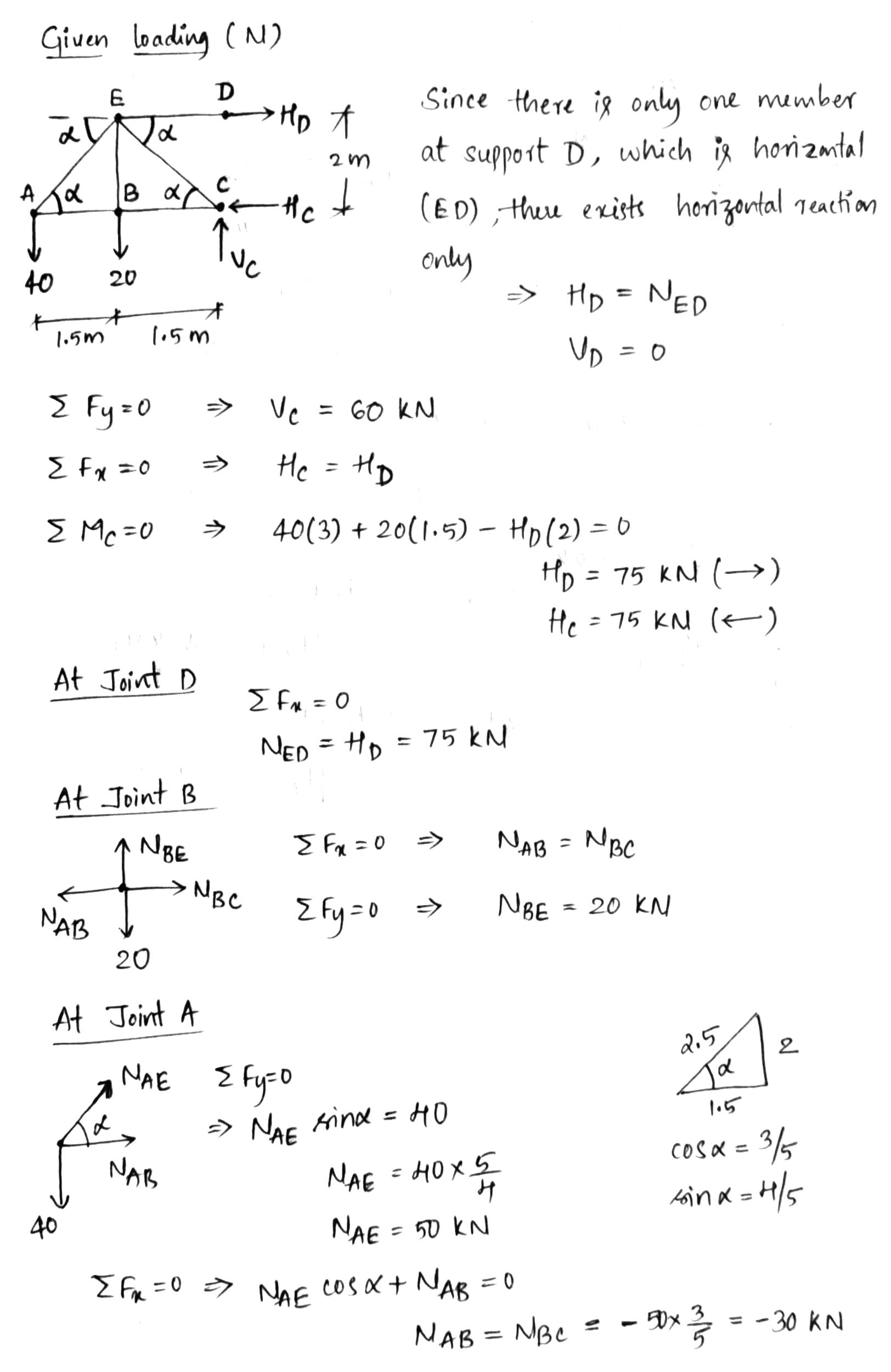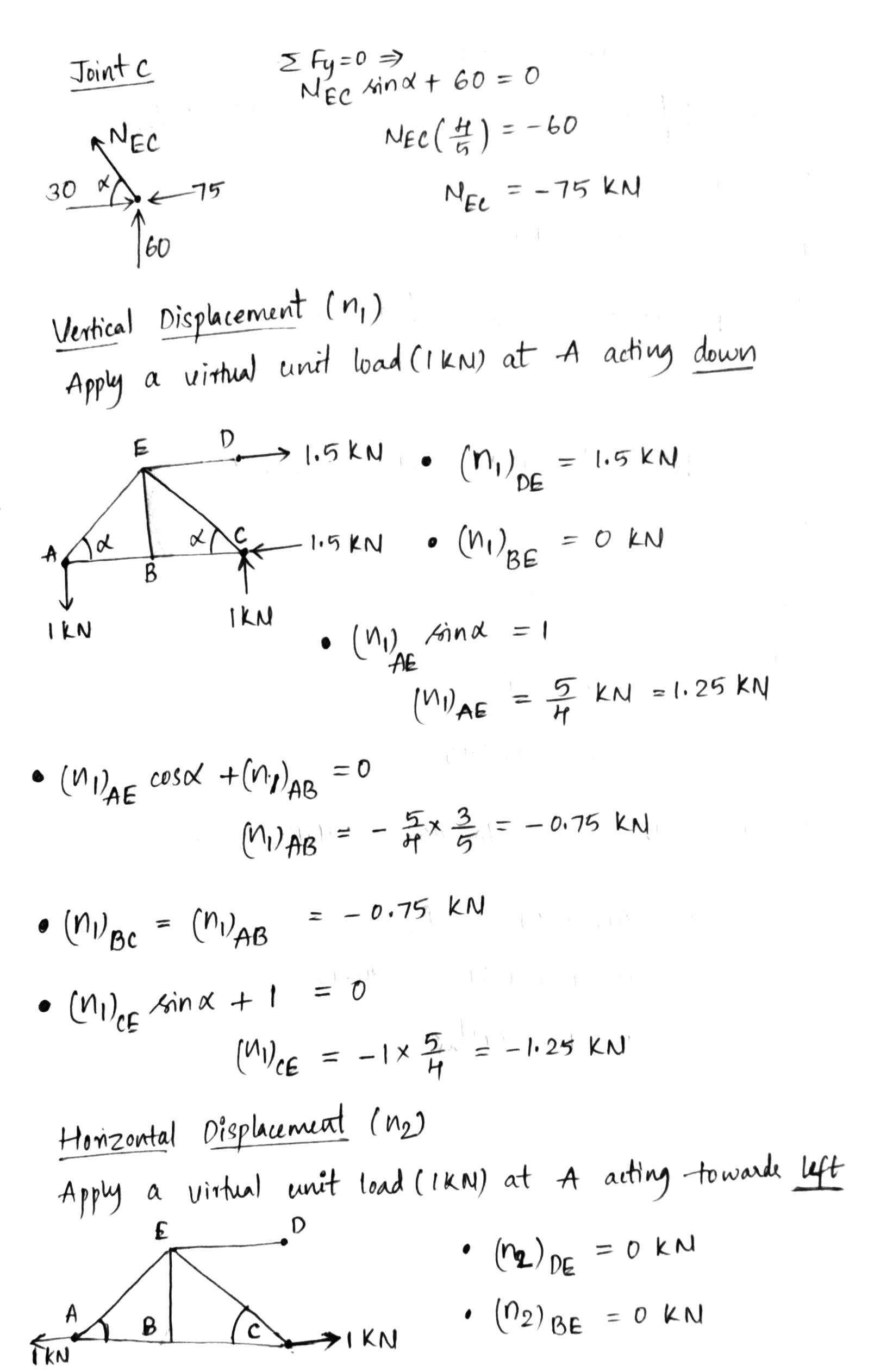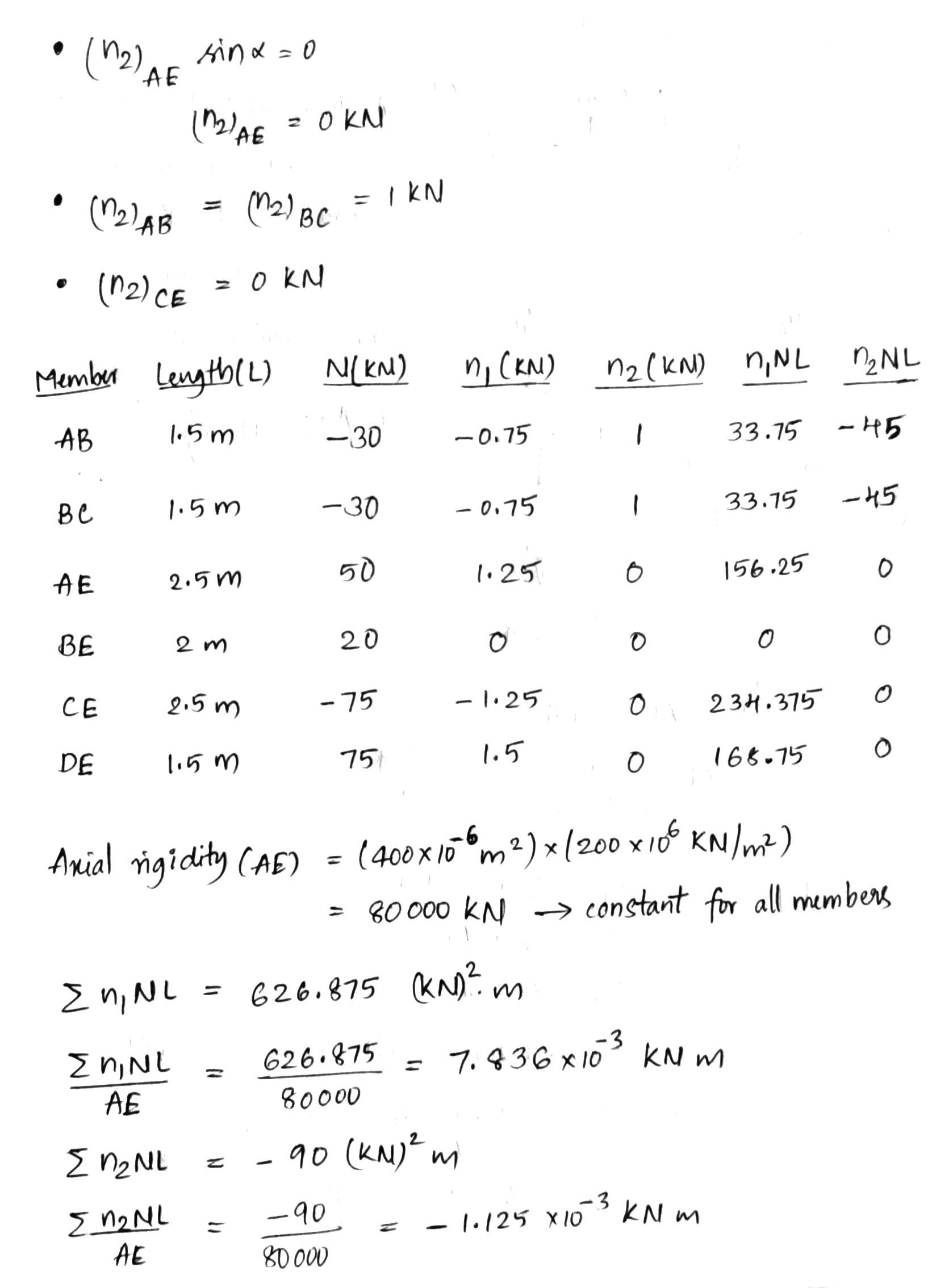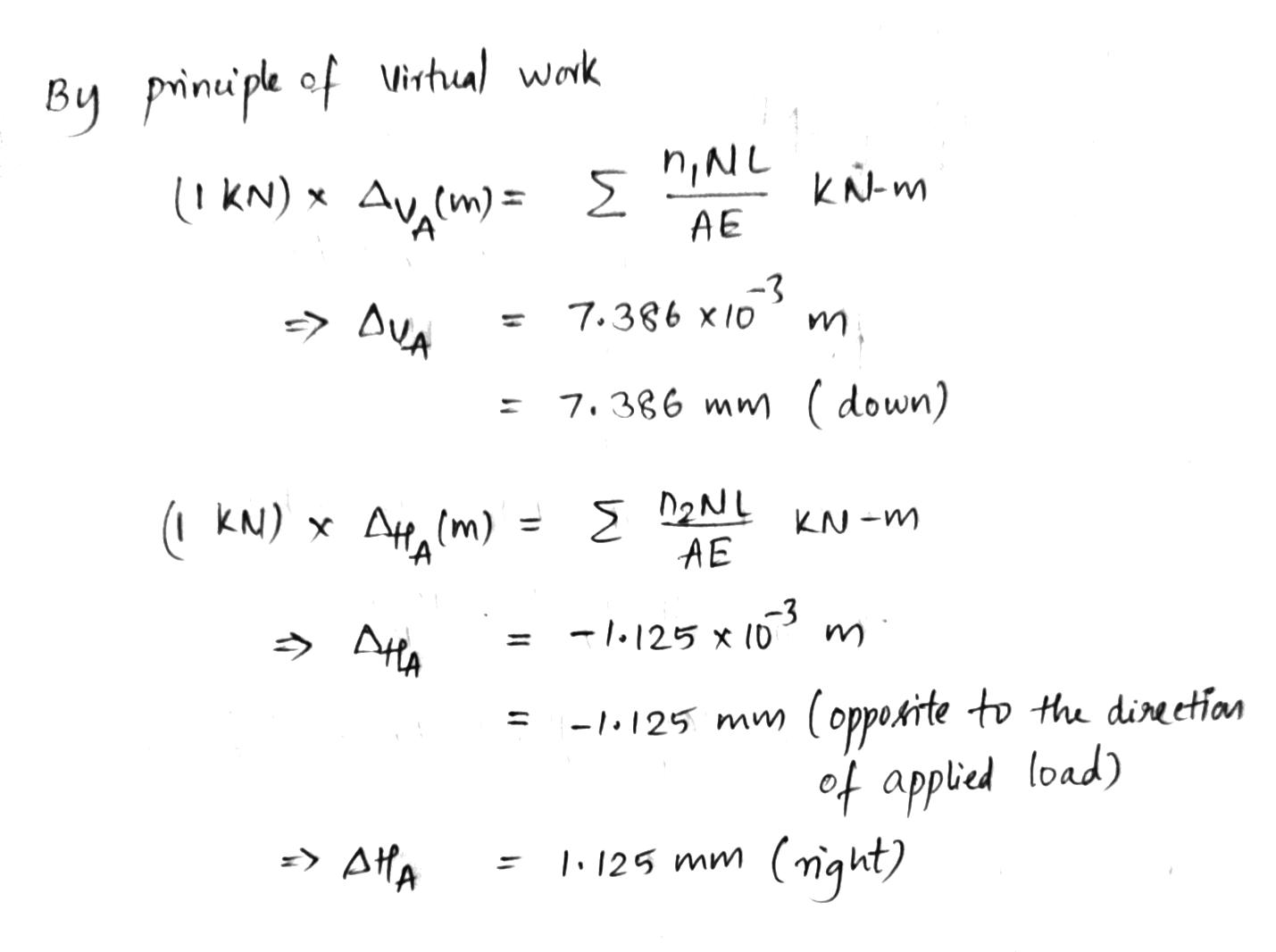#### Earn Coin

Coins can be redeemed for fabulous gifts.

Similar Homework Help Questions
• ### Q3: Determine the vertical and horizontal displacement of joint A for the truss shown in Fig....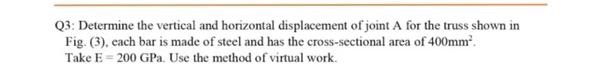Q3: Determine the vertical and horizontal displacement of joint A for the truss shown in Fig. (3). each bar is made of steel and has the cross-sectional area of 400mm Take E = 200 GPa Use the method of virtual work. E D 2 m |в -1.5 m -1.5 m 20 KN 40 KN Fig. (3)

• ### i need the answer quickly fast as you can Q3: Determine the vertical and horizontal displacement...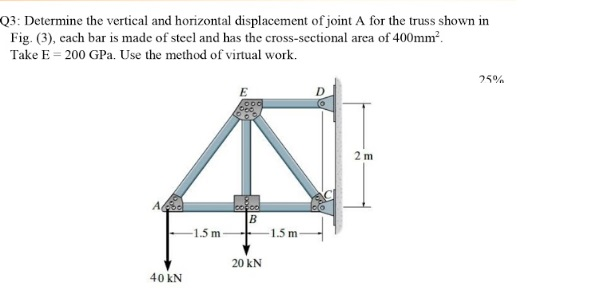i need the answer quickly fast as you can Q3: Determine the vertical and horizontal displacement of joint A for the truss shown in Fig. (3), cach bar is made of steel and has the cross-sectional area of 400mm? Take E = 200 GPa. Use the method of virtual work. 25% E 2 m А. cool B 1.5 m -1.5 m 20 KN 40 KN

• ### Solve It fast Please Q3: Determine the vertical and horizontal displacement of joint A for the...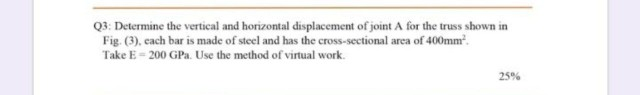Solve It fast Please Q3: Determine the vertical and horizontal displacement of joint A for the truss shown in Fig. (3). cach bar is made of steel and has the cross-sectional area of 400mmº. Take E = 200 GP. Use the method of virtual work. 25% E OO D 2 m AAS ered B -1.5 m -1.5 m 20 KN 40 KN Fig. (3)

• ### stion 1 Determine the total vertical displacement of joint A (due to the load and tempreature...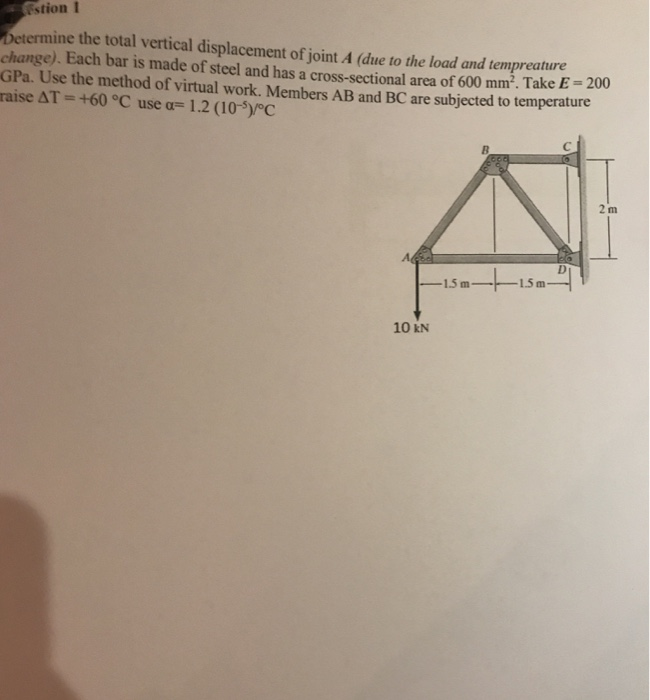stion 1 Determine the total vertical displacement of joint A (due to the load and tempreature change). Each bar is made of steel and has a cross-sectional area of 600 mm². Take E - 2001 GPa. Use the method of virtual work. Members AB and BC are subjected to temperature raise AT = +60 °C use a= 1.2 (10-5)/°C —15 m- 1.5m 10 KN

• ### 15 m B- Question 3: Each member of the truss shown is made of steel (E- 2 1 0 GPa ) and has a cro...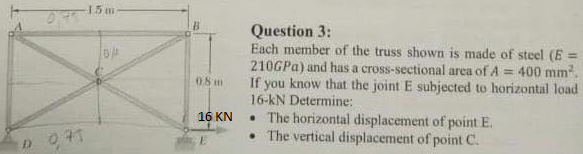15 m B- Question 3: Each member of the truss shown is made of steel (E- 2 1 0 GPa ) and has a cross-sectional area of A If you know that the joint E subjected to horizontal load 16-kN Determine: .The horizontal displacement of point E . The vertical displacement of point C. 400 mm2 0.8m 16 KN 15 m B- Question 3: Each member of the truss shown is made of steel (E- 2 1 0 GPa )...

• ### 2. Determine the vertical displacement at joint B and horizontal displacement at joint D using Castigliano's...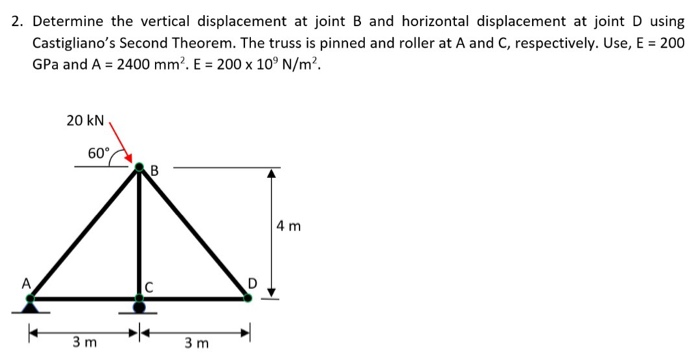2. Determine the vertical displacement at joint B and horizontal displacement at joint D using Castigliano's Second Theorem. The truss is pinned and roller at A and C, respectively. Use, E = 200 GPa and A = 2400 mm. E = 200 x 10°N/m². 20 KN 60 CAB 4 m 3m 3m

• ### 14–81. Determine the horizontal displacement of jou on the truss. Each A992 steel member has a...14–81. Determine the horizontal displacement of jou on the truss. Each A992 steel member has a cross-sect area of 1935 mm. Splacement of joint C 14-82. Determine the horizontal displacement of joint B on the truss. Each A992 steel member has a cross-sectional area of 1935 mm. 10 KN C 75 kN 1.2 m obdo be255 zil bado Todo IA a D - 0.9 m- Probs. 14-81/82

• ### The three-bar truss ABC shown in the figure has a span L = 3 m and...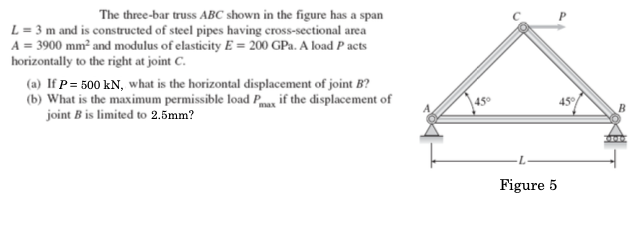The three-bar truss ABC shown in the figure has a span L = 3 m and is constructed of steel pipes having cross-sectional area A = 3900 mm and modulus of elasticity E = 200 GPa. A load P acts horizontally to the right at joint C. (a) If P= 500 kN, what is the horizontal displacement of joint B? (b) What is the maximum permissible load Pox if the displacement of joint B is limited to 2.5mm? 45 45°...

• ### oblem 2 etermi ine the vertical displacement at joint A. Each bar is made of eel...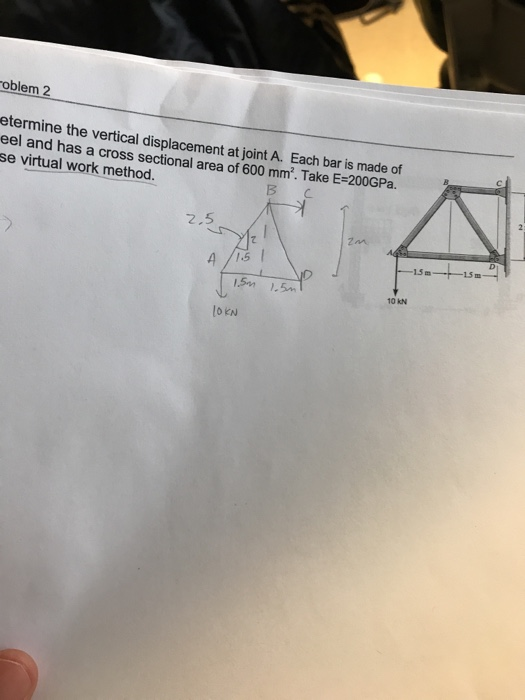oblem 2 etermi ine the vertical displacement at joint A. Each bar is made of eel and has a cross sectional area of 600 mm. Take E-200GPa. se virtual work method. ВС 2.5 15m 15 m 10 kN OKN

• ### The cross-sectional area of each member of the truss shown in the figure is 4 =...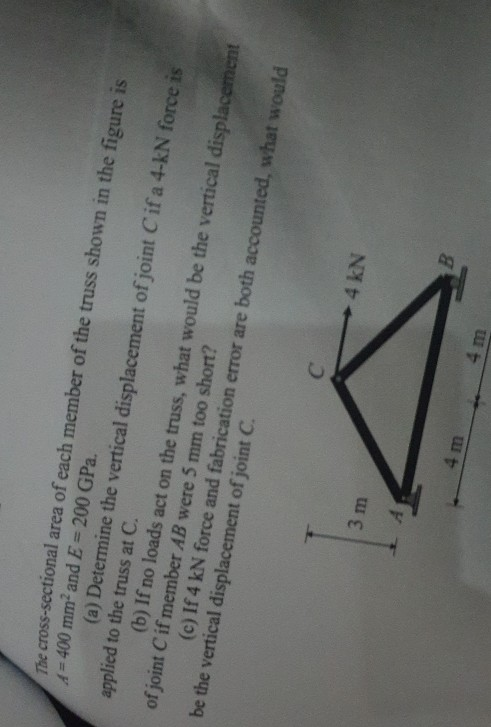The cross-sectional area of each member of the truss shown in the figure is 4 = 400 mm and E = 200 GPa. (a) Determine the vertical displacement of joint Cif a 4-KN force is applied to the truss at C. (b) If no loads act on the truss, what would be the vertical displacement of joint C if member AB were 5 mm too short? (c) If 4 kN force and fabrication error are both accounted, what would be...

Free Homework App